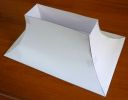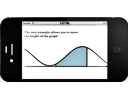Search IntMath
Close

450+ Math Lessons written by Math Professors and Teachers

5 Million+ Students Helped Each Year

1200+ Articles Written by Math Educators and Enthusiasts

Simplifying and Teaching Math for Over 23 Years

# IntMath Newsletter: Curved surface solution, Mathapedia

By Murray Bourne, 10 Apr 2014

10 Apr 2014

1. Solution for the range hood problem
2. Resource - Mathapedia
3. IntMath polls
4. Math puzzles
5. Friday math movie - 8 math talks to blow your mind
6. Final thought - Despicable

## 1. Range hood curved sides problemLast newsletter I posed a problem regarding a stove range hood with curved sides. I usually get about 10 responses to each puzzle, but this time there was just one reply, which said "It's very hard!". Here's my go at a solution. My first attempts were to make it much more complicated than it needs to be. Range hood curved sides solution

## 2. MathapediaMathapedia is an interesting devlopment by Dan Lynch. It's a system for developing online math documents, and it produces output for all sorts of devices, including tablets and phones. It easily produces interactive math documents, like this example: Interactive (run your mouse over any of the diagrams on that page). There's also an interesting section on The Art of Digital Publishing.

## 3. IntMath poll - good teaching

What's the main thing teachers should do so you learn better?

There is some good advice to follow here, especially for math teachers. Obviously, there are not many friendly math classes out there! Here are the results.

Create a warm and friendly classroom: 26%

Be enthusiastic: 24%

Be flexible: 21%

Love learning: 11%

Respect students more: 11%

Set high expectations: 8%

Poll date: Feb - Apr 2014

Latest poll: The current poll asks what kind of math you will find most useful in the future. You can respond on any (inner) page of IntMath.

## 4. Math puzzles

New math puzzle

For integers A, B, how many solutions (A, B) are there for

A2 + B2 ≤ 12?

## 5. Math movies - 8 math talks to blow your mind

There are some really good thought provoking videos here. I've featured some of them in the past. I hope you find something to blow your mind!TED has an incredibly broad range of really good talks. This collection of provocative math talks has been around for a while now, but they're still worth a look. 8 math talks to blow your mind

## 6. Final thought: Despicable

Here's a great quote from Rockefeller, 19th century billionaire businessman and philanthropist. He was also founder of both the University of Chicago and Rockefeller University and funded the establishment of Central Philippine University in the Philippines. [Source]

I know of nothing more despicable and pathetic than a man who devotes all the hours of the waking day to the making of money for money's sake. [John D. Rockefeller]

Until next time, enjoy whatever you learn.

1. Peter Hunter says:

(-3,0),(-3,1),(-3,-1),
(-2,-2),(-2,-1),(-2,0),(-2,1),(-2,2),
(-1,-3)(-1,-2),(-1,-1),(-1,0),(-1,1),(-1,2),(-1,3),
(0,-3),(0,-2),(0,-1),(0,0),(0,1),(0,2),(0,3),
(1,-3)(1,-2),(1,-1),(1,0),(1,1),(1,2),(1,3),
(2,-2),(2,-1),(2,0),(2,1),(2,2),
(3,0),(3,1),(-3,-1)

37sets

2. Peter Hunter says:

What kind of math for the future? Statistics. People need.to evaluate good claims from bad when proponents argue with statistics.

3. Peter Hunter says:

This is a follow up to my original answer to the puzzle. The answer is still 37 sets, but a better explanation is due.

First, integers are natural numbers which include negatives, zero and positive whole numbers.

Second, the squared value of any one number cannot exceed 12, so that limits the range of A and B to [-3,3]

Third, to get the number of sets over the range [-3,3] is easy because you just have to find the negative (or positive) sets that satisfy the condition, double it (because the opposite sign value will also satisfy the condition because they are squared) and add 1 for zero.

For example, take A=-3, the negative sets that satisfy the condition only includes (-3,-1) or 1 set. Double that for the positive side (-3,1) and add 1 for the zero set (-3,0), making a total of 3 sets.

Do this for each integer on the negative (or positive) side. I already showed A=-3 yields 3 sets. Because of the symetery of squares, you A=+3 also yields 3 sets.

So one can build up a simple table:
A-value      Sets
-3             3
-2             5
-1             7
Total         15

Double this for integers in the positive range [1,2,3], so we are up to 30 sets.

Zero yields valid values for all intergers in the range [-3,3] or 7, giving a total of 37 sets.

4. Peter Hunter says:

Murray,
Two comments. First its interesting the difference in answers based on the device used to submit. My first response was done on an iPad. I find it hard to be wordy on that medium. The second was done on my computer with a full keyboard. That would be an interesting survey question: Are you more thoughtful (and complete) when providing an answer on a smartphone, tablet, computer, or pen and paper?

Second, it would be really nice if in addition to the xhtml codes you permit, one could also use and

5. frank di nunno says:

I am glad I somehow got the intmath newsletter, we got a

new computer and I lost the site. frank

6. Emily says:

(-3,-1),(-3,0),(-3,1)
(-2,-2),(-2,-1),(-2,0),(-2,1),(-2,2)
(-1,-3),(-1,-2),(-1,-1),(-1,0),(-1,1),(-1,2),(-1,3)
(0,-3),(0,-2),(0,-1),(0,0),(0,1),(0,2),(0,3)
(1,-3),(1,-2),(1,-1),(1,0),(1,1),(1,2),(1,3)
(2,-2),(2,-1),(2,0),(2,1),(2,2)
(3,-1),(-3,0),(-3,1)

Total 37 solutions

7. James V says:

POSSIBLE SOLUTIONS:

TOTAL NUMBER OF POSSIBLE SOLUTION IS :25

case1: If A=0,B=0, then A^2+B^2 <=12 NO OF SOLUTION=01

Case2: If A=1, then B= 0,1,2,3 : NO OF SOLUTIONS=4

Case3: If A=-1, then B=0,1,2,3 : NO OF SOLUTIONS=4

Case4: IF A=1, THEN B=-1,-2,-3: NO OF SOLUTION=3

Case5: If A=2, THEN B=0,1,2:NO OF SOLUTIONS: 3

CASE6: IF A=-2,THEN B=0,1,2 ; NO OF SOLUTIONS=3

CASE7: IF A =2, THEN B=-1,-2; NO OF SOLUTIONS=2

CASE8: IF A=3, THEN B=0,1; NO OF SOLUTIONS=2

CASE9: IF A=-3, THEN B=0,1; NO OF SOLUTIONS=2

CASE10: IF A=3, THEN B=-1; NO OF SOLUTIONS=1

8. Tomas Garza says:

There are 37 integral solutions {A, B}, viz.

{-3, -1}, {-3, 0}, {-3, 1}, {-2, -2}, {-2, -1}, {-2, 0}, {-2, 1}, {-2, 2}, {-1, -3}, {-1, -2}, {-1, -1}, {-1, 0}, {-1, 1}, {-1, 2}, {-1, 3}, {0, -3}, {0, -2}, {0, -1}, {0, 0}, {0, 1}, {0, 2}, {0, 3}, {1, -3}, {1, -2}, {1, -1}, {1, 0}, {1, 1}, {1, 2}, {1, 3}, {2, -2}, {2, -1}, {2, 0}, {2, 1}, {2, 2}, {3, -1}, {3, 0}, {3, 1}

This is readily obtained using Mathematica's NSolve function:

NSolve[a^2 + b^2 <= 12, {a, b}, Integers]

9. Francis Kisner says:

A^2 + B^2 ≤ 12 for A and B integers
Setting B = {-1, 0, 1}, we can have A = {-3, -2, -1, 0, 1, 2, 3} That is 21 possible sets
For B = {-2, 2} A = {-2, -1, 0, 1, 2} This adds 10 sets
For B = {-3, 3} A = {-1, 0, 1} And here we have another 6 sets
|A| or |B| cannot exceed 3 so we have all the possible sets covered here
21 + 10 + 6 = 37

10. Nicos Mavrommatis says:

The pairs (A,B) are: (0,0),(0,1),(0,2),(0,3),(1,0),(1,1),(1,2),(1,3),(2,0),(2,1),(2,2),(3,0),(3,1),(0,-1),(0,-2),(0,-3),(-1,0),(-1,1),(1,-1),(-1,-1),(-1,2),(1,-2),(-1,-2),(-1,3),(1,-3),(-1,-3),(-2,0),(-2,1),(2,-1),(-2,-1),(-2,2),(2,-2),(-2,-2),(-3,0),(-3,1),(3,-1),(-3,-1) a total of 37 pairs.

11. Cichy says:

Use of Geogebra:
inequality x^2 + y^2 <= 12 gives a circle with circumfarance

Calculating points in different areas of a a circle
x-axis and y-axis: 2x6 = 12
Point of origin: 1
4x6 = 24

Total= 37

12. Cichy says:

Geogebra: inequality x^2 + y^2 <= 12.
Result: a circle with circumference

Counting points:

axes:
2x6=12
point of origin 1

Total: 12+1+24=37

13. John says:

37 Solutions in total
A is 0, B can be 0, +/-1, +/-2, +/-3 7 solutions
A is +/-1 B can be 0, +/-1, +/-2, +/-3 14 solutions
A is +/-2 B can be 0, +/-1, +/-2 10 solutions
A is +/-3 B can be 0, +/-1 6 solutions

14. mohamed says:

there are (4) solutions for

ِِA^2 + B^2 ≤ 12

(1,1)
(1,2)
(2,1)
(2,2)

### Comment Preview

HTML: You can use simple tags like <b>, <a href="...">, etc.

To enter math, you can can either:

1. Use simple calculator-like input in the following format (surround your math in backticks, or qq on tablet or phone):
a^2 = sqrt(b^2 + c^2)
(See more on ASCIIMath syntax); or
2. Use simple LaTeX in the following format. Surround your math with $$ and $$.
$$\int g dx = \sqrt{\frac{a}{b}}$$
(This is standard simple LaTeX.)

NOTE: You can mix both types of math entry in your comment.